Share

# Frank solutions for Class 10 Maths chapter 14 - Symmetry

#### Frank Frank Class 10 Mathematics Part 2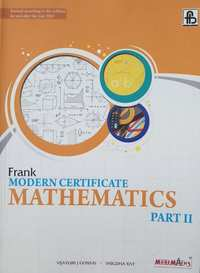## Chapter 14: Symmetry

Exercise 14.1

#### Frank solutions for Class 10 Maths Chapter 14 Exercise Exercise 14.1 [Page 0]

Construct an isosceles triangle whose equal sides are 7 cm each and the base side is 5 cm. Draw all its lines of symmetry.

Take a graph paper and mark the points A(2,0), B(2,8) and C(S,4) on it. Taking AB as the line of symmetry, obtain and write the co-ordinates of point D. Complete the quadrilateral ABCD and give its geometrical name.

Take a graph paper and mark the points P(2, 1), Q(7, 1) and R(7,5). Taking QR as the line of symmetry, obtain and write the co-ordinates of point S.

A(8,2) and B(6,4) are the vertices of a figure which is symmetrical about x = 6 and y = 2. Complete the figure and give the geometrical name of the figure.

A(2,2) and B(5,5) are the vertices of a figure which is symmetrical about xaxis. Complete the figure and give its geometrical name.

A( 4, 1 ), B(2,3) and C( 5,6) are the vertices of a figure which is symmetrical about x=7. Complete the figure and give the geometrical name of the figure if any.

In the following figure, the line of symmetry has been drawn with a dotted line. Identify the corresponding sides and the corresponding angles about the line of symmetry.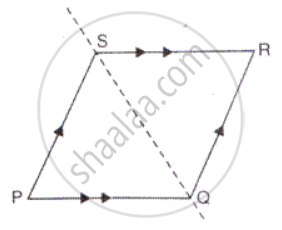In the following figures, the line of symmetry has been drawn with a dotted line. Identify the corresponding sides and the corresponding angles about the line of symmetry.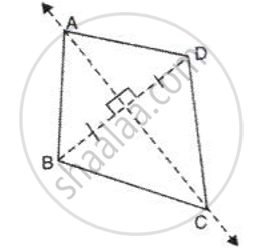In the following figures, the line of symmetry has been drawn with a dotted line. Identify the corresponding sides and the corresponding angles about the line of symmetry.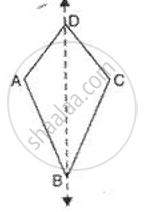In the following figures, the line of symmetry has been drawn with a dotted line. Identify the corresponding sides and the corresponding angles about the line of symmetry.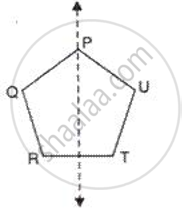Draw the line of symmetry of the following figure and also mark their points of symmetry, if any: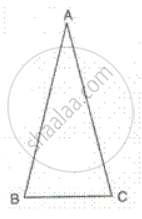Draw the line of symmetry of the following figure and also mark their points of symmetry, if any: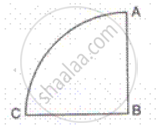Draw the line of symmetry of the following figure and also mark their points of symmetry, if any:Draw the line of symmetry of the following figure and also mark their points of symmetry, if any: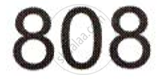Complete the following figure, about the given lines of symmetry which are shown by dotted lines and name the figure thus obtained: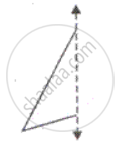Complete the following figure, about the given lines of symmetry which are shown by dotted lines and name the figure thus obtained: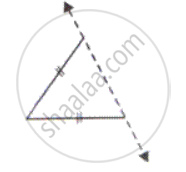Complete the following figure, about the given lines of symmetry which are shown by dotted lines and name the figure thus obtained: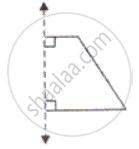Complete the following figure, about the given lines of symmetry which are shown by dotted lines and name the figure thus obtained: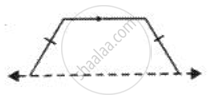Complete the following figure, about the given lines of symmetry which are shown by dotted lines and name the figure thus obtained: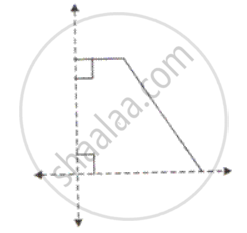Complete the following figure, about the given lines of symmetry which are shown by dotted lines and name the figure thus obtained: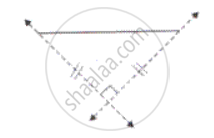Complete the following figure, about the given lines of symmetry which are shown by dotted lines and name the figure thus obtained: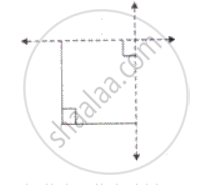Complete the following figure, about the given lines of symmetry which are shown by dotted lines and name the figure thus obtained: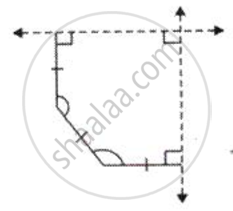Complete the following figure, about the given lines of symmetry which are shown by dotted lines and name the figure thus obtained: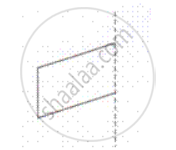Complete the following figure, about the given lines of symmetry which are shown by dotted lines and name the figure thus obtained: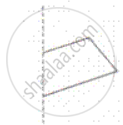Complete the following figure, about the given lines of symmetry which are shown by dotted lines and name the figure thus obtained: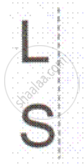Complete the following figure, about the given lines of symmetry which are shown by dotted lines and name the figure thus obtained: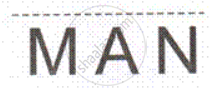Given below are part of a geometrical figure. Complete the figure so that both axes are lines of symmetry.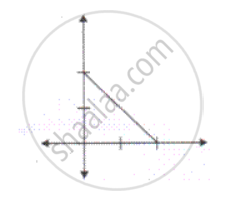Given below are part of a geometrical figure. Complete the figure so that both axes are lines of symmetry.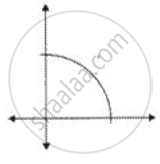Given below are part of a geometrical figure. Complete the figure so that both axes are lines of symmetry.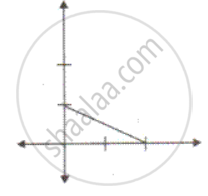Given below are part of a geometrical figure. Complete the figure so that both axes are lines of symmetry.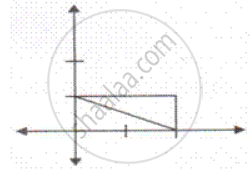Construct a triangle ABC in which each side measures 5.8 cm. Draw all the possible lines of symmetry.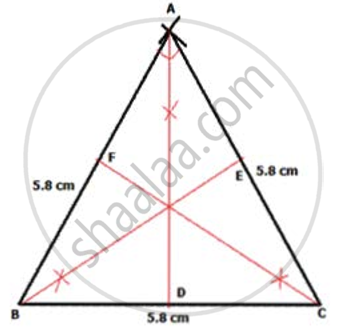Construct a paralIelogra m PQRS in wh i di QR = 5. 4 cm , SR = 6. 0 cm and ∠ Q= 60°. Draw its lines of symmetry, if possible.

Construct a square of side 4.8 cm and draw all its lines of symmetry .

Construct a regular hexagon of side = 3.8 cm and draw all its lines of symmetry .

Construct a rhombus ABCD with AB= 5.0 cm and AC= 8 cm. Draw its lines of symmetry.

Construct an isosceles right-angled triangle, having hypotenuse= 8 cm. Draw its lines of symmetry.

Construct a Δ ABC in whidi BA= BC= 6 cm and AC= 4.5 cm. Taking AC as line of symmetry, obtain a point D to form a quadrilateral ABCD. Name the figure ABCD.

Construct a Δ PQR in which ∠ R = 90 ° ,  PQ = 5.2 cm and QR= 2.6 cm. Complete the figure taking PR as the line of symmetry and name the figure.

## Chapter 14: Symmetry

Exercise 14.1

#### Frank Frank Class 10 Mathematics Part 2## Frank solutions for Class 10 Mathematics chapter 14 - Symmetry

Frank solutions for Class 10 Maths chapter 14 (Symmetry) include all questions with solution and detail explanation. This will clear students doubts about any question and improve application skills while preparing for board exams. The detailed, step-by-step solutions will help you understand the concepts better and clear your confusions, if any. Shaalaa.com has the CISCE Frank Class 10 Mathematics Part 2 solutions in a manner that help students grasp basic concepts better and faster.

Further, we at Shaalaa.com provide such solutions so that students can prepare for written exams. Frank textbook solutions can be a core help for self-study and acts as a perfect self-help guidance for students.

Concepts covered in Class 10 Mathematics chapter 14 Symmetry are Symmetry Examples, Lines of Symmetry.

Using Frank Class 10 solutions Symmetry exercise by students are an easy way to prepare for the exams, as they involve solutions arranged chapter-wise also page wise. The questions involved in Frank Solutions are important questions that can be asked in the final exam. Maximum students of CISCE Class 10 prefer Frank Textbook Solutions to score more in exam.

Get the free view of chapter 14 Symmetry Class 10 extra questions for Maths and can use Shaalaa.com to keep it handy for your exam preparation

S